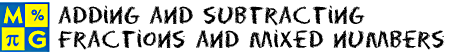# Adding and Subtracting Fractions and Mixed NumbersThis page lists the Learning Objectives for all lessons in Unit 16.

#### Adding Fractions with Like Denominators

The student will be able to:

• Define units, common denominator, simplify, and lowest terms
• Describe the procedure for adding fractions with like denominators.
• Determine the sum of two or more fractions with like denominators by applying the procedure above.
• Simplify the result when necessary.
• Recognize that only the numerators should be added, not the denominators.
• Apply addition procedures to complete five interactive exercises.

#### Subtracting Fractions with Like Denominators

The student will be able to:

• Define fraction bar.
• State the Multiplication Property of Zero.
• State the additive inverse property of zero.
• Describe the procedure for subtracting fractions with like denominators.
• Find the difference of two fractions with like denominators by applying the procedure above.
• Simplify the result when necessary.
• Recognize that a fraction bar indicates that a division of the numerator by the denominator will be performed
• Recognize that division by zero is undefined.
• Recognize that any fraction with zero in the numerator and a nonzero number in the denominator equals zero.
• Apply subtraction procedures to complete five interactive exercises.

#### Adding and Subtracting Fractions with Unlike Denominators

The student will be able to:

• Define least common denominator (LCD), least common multiple, and equivalent fractions.
• Find a common denominator by multiplying the denominators together.
• Rename fractions using their least common denominator.
• Identify the LCD of two or more fractions.
• Add fractions with unlike denominators by converting to equivalent fractions with a LCD.
• Simplify the result when necessary.
• Recognize that the numerator and the denominator of a fraction must be multiplied by the same nonzero whole number in order to have equivalent fractions.
• Recognize that there are two methods for adding and subtracting fractions with unlike denominators: common denominators and LCD.
• Describe the procedure for each method of adding and subtracting fractions with unlike denominators.
• Add and subtract fractions with unlike denominators by applying one the procedures above.
• Apply procedures to complete five interactive exercises.

The student will be able to:

• Define mixed numbers.
• Describe the procedure for adding mixed numbers.
• Recognize that a mixed number consists of a whole-number part and a fractional part.
• Recognize that it is easier to arrange the work vertically in order to add mixed numbers.
• Find the sum of two mixed numbers by applying the procedure for adding mixed numbers.
• Find the sum of a mixed number and a whole number by applying the procedure for adding mixed numbers.
• Simplify the result when necessary.
• Apply the procedure to complete five interactive exercises.

#### Subtracting Mixed Numbers

The student will be able to:

• Identify the whole-number part and the fractional part of a mixed number.
• Describe the procedure for subtracting mixed numbers with like denominators.
• Borrow a mixed number by converting it to an improper fraction.
• Describe the procedure for subtracting mixed numbers with unlike denominators.
• Subtract mixed numbers with like and unlike denominators by following the procedures presented.
• Subtract a mixed number from a whole number by following the procedures presented.
• Subtract a whole number from a mixed number by following the procedures presented.
• Recognize that there is another way to subtract mixed numbers is to convert each mixed number into an improper fraction.
• Recognize subtract mixed numbers by convert each mixed number into an improper fraction can lead to careless errors.
• Solve a real-world problem by subtracting mixed numbers.
• Apply procedures to complete five interactive exercises.

### Solving Word Problems

The student will be able to:

• Examine problems involving addition and subtraction of fractions and mixed numbers.
• Identify strategies for solving each problem.
• Apply strategies for solving each problem.
• Connect addition and subtraction of fractions and mixed numbers with the real word.
• Apply all concepts and procedure to complete five interactive exercises with real-world problems.

#### Practice Exercises

The student will be able to:

• Examine ten interactive exercises for all topics in this unit.
• Identify the concepts and procedures needed to complete each practice exercise.
• Compute all answers and solve all problems by applying appropriate concepts and procedures.
• Self-assess knowledge and skills acquired from the instruction provided in this unit.

#### Challenge Exercises

The student will be able to:

• Evaluate ten challenging exercises for all topics in this unit.
• Analyze each problem to identify the given information.
• Formulate a strategy for solving each problem.
• Apply strategies to solve problems and write answers.
• Synthesize all information presented in this unit.
• Develop strong problem-solving skills and the ability to handle challenging problems.

#### Solutions

The student will be able to:

• Examine the solution for each exercise presented in this unit.
• Compare solutions to completed exercises.
• Identify which solutions need to be reviewed.
• Identify and evaluate incorrect answers to exercises from this unit.
• Amend and label original answers.
• Identify areas of strength and weakness.
• Decide which concepts and procedures need to be reviewed from this unit.

### Order your CD today for Home or SchoolOR